Refer to our Texas Go Math Grade 1 Answer Key Pdf to score good marks in the exams. Test yourself by practicing the problems from Texas Go Math Grade 1 Lesson 6.7 Answer Key Use Make a 10 to Add.

Explore
Draw to show the addends. Then draw to show how to make a ten. Write the sum.For The Teacher

• Read the following problem. Sean has 6 big paper clips and 7 small paper clips. How many paper clips does he have? Ask children to draw counters in the ten frames to show how to solve by making a ten.
Explanation:Math Talk
Mathematical Processes
Describe how the drawings show how to make a ten to solve 6 + 7.
Explanation:
6 + 7
breaking 7 as 4 and 3
6 + 4 = 10
10 + 3 = 13
6 + 7 = 13

Model and Draw

What is 9 + 6?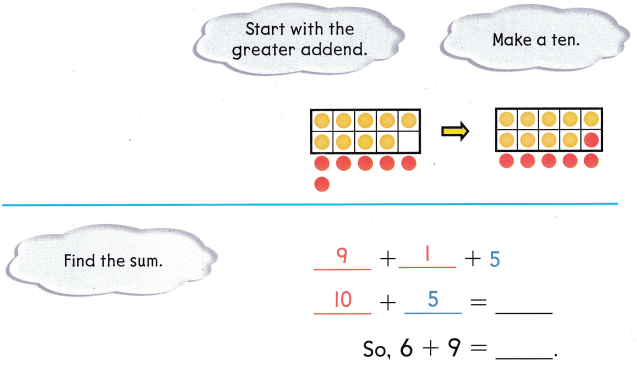Explanation:
9 + 6
Breaking 6 as 1 and 5
9 + 1 = 10
10 + 5 = 15
so, 9 + 6 = 15

Share and Show

Write to show how you make a ten. Then add.

Question 1.
What is 8 + 4?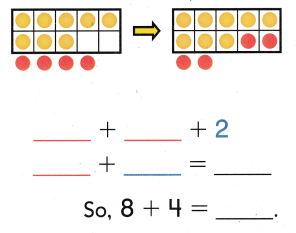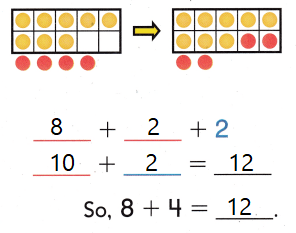Explanation:
8 + 4
Breaking 4 as 2 and 2
8 + 2 = 10
10 + 2 = 12
8 + 4 = 12

Question 2.
What is 5 + 7?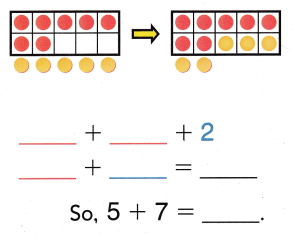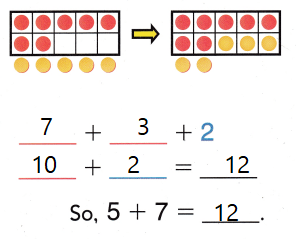Explanation:
5 + 7
breaking 5 as 3 and 2
7 + 3 = 10
10 + 2 = 12
5 + 7 = 12

Problem Solving

H.O.T. Write to show how you make a ten. Then add.Question 3.
What is 7 + 8?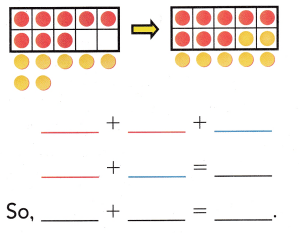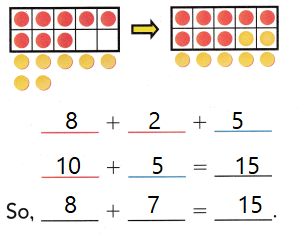Explanation:
7 + 8
Breaking 7 as 2 and 5
8 + 2 = 10
10 + 5 = 15
7 + 8 = 15

Question 7.
What is 9 + 8?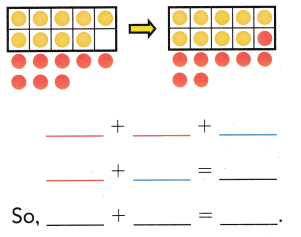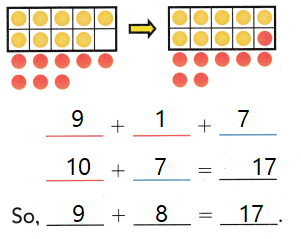Explanation:
9 + 8
Breaking 8 as 1 and 7
9 + 1 = 10
10 + 7 = 17
9 + 8 = 17

Multi-Step Use the model. Write to show how you make a ten. Then add.

Question 5.
Leah has 8 green balls of clay. Joe has 6 blue balls of clay. How many balls of clay do they have?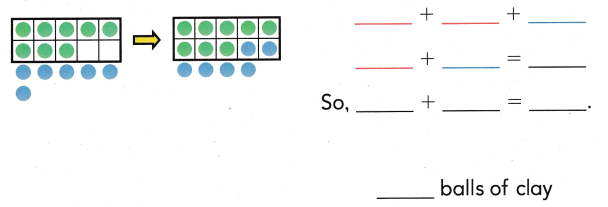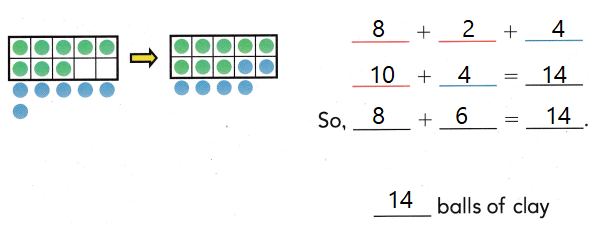Explanation:
Leah has 8 green balls of clay. Joe has 6 blue balls of clay.
8 + 6
Breaking 6 as 2 and 4
8 + 2 = 10
10 + 4 = 14
8 + 6 = 14
14  balls of clay  they have

Question 6.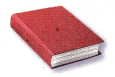(A) 12
(B) 13
(C) 14
Explanation:
8 + 6 = 14
14 books that Kan and Ryan read

Question 7.
Apply Connor has 7 red hens. He has 5 white hens. How many hens does Connor have?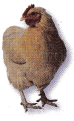(A) 12
(B) 11
(C) 13
Explanation:
Connor has 7 red hens. He has 5 white hens.
7 + 5 = 12
12 hens that Connor have

Question 8.
Multi-Step There are 9 ants on the hill. 3 more ants join them. Then 2 ants walk away. How many ants are on the hill now?
(A) 12
(B) 10
(C) 7Explanation:
There are 9 ants on the hill. 3 more ants join them.
9 + 3 = 12
Then 2 ants walk away.
12 – 2 = 10
10 ants are on the hill now

Question 9.
Texas Test Prep Which way shows how to make a ten to solve 9 + 7?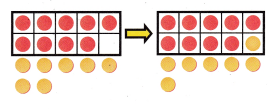(A) 7 + 3 + 9
(B) 9 + 1 + 6
(C) 9 + 1 + 7
Explanation:
9 + 7
Breaking 7 as 1 and 6
9 + 1 = 10
10 + 6 = 16

Take Home Activity

• Have your child show you how to make a ten to solve 8 + 7.
Explanation:
8 + 7
Breaking 7 as 2 and 5
8 + 2 = 10
10 + 5 = 15
8 + 7  = 15

### Texas Go Math Grade 1 Lesson 6.7 Homework and Practice Answer Key

Write to show how you make a ten. Then add.

Question 1.
What is 6 + 9?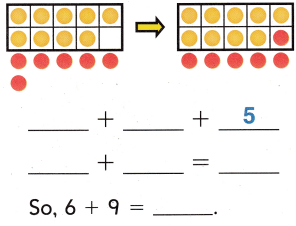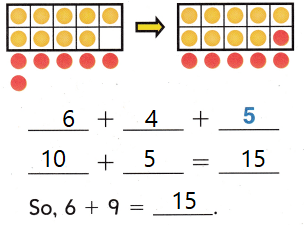Explanation:
6 + 9
Breaking 9 as 4 and 5
6 + 4 = 10
10 + 5 = 15

Question 2.
What is 7 + 6?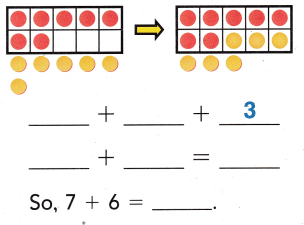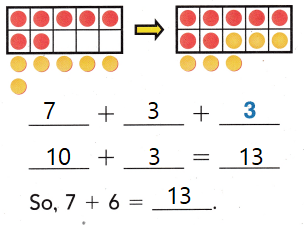Explanation:
7 + 6
Breaking 6 as 3 and 3
7 + 3 = 10
10 + 3 = 13

Problem Solving

Use the model. Write to show how you make a ten. Then add.

Question 3.
A bear ate 5 fish. Another bear ate 9 fish. How many fish did the bears eat?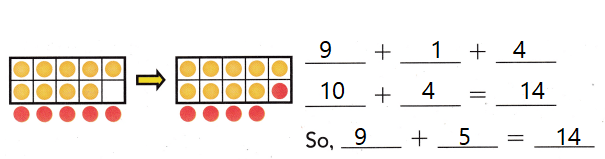Explanation:
A bear ate 5 fish. Another bear ate 9 fish.
9 + 5
Breaking 5 as 1 and 4
9 + 1 = 10
10 + 4 = 14
14 fish that the bears eat

Texas Test Prep

Lesson Check

Question 4.
9 bees fly around the hive. Then 3 more bees join them. How many bees fly around the hive?(A) 13
(B) 12
(C) 10
Explanation:
9 bees fly around the hive. Then 3 more bees join them.
9 + 3 = 12
12 bees fly around the hive

Question 5.
A chipmunk ate 7 seeds. Then she ate 9 more seeds. How many seeds did the chipmunk eat?(A) 17
(B) 15
(C) 16
Explanation:
A chipmunk ate 7 seeds.
Then she ate 9 more seeds.
9 + 7 = 15
15 seeds that the chipmunk eat

Question 6.
Multi-Step Kai put 8 sheets of paper in his notebook. Then he put ¡n 6 more sheets of paper. Then he took out 2 sheets of paper. How many sheets of paper are in Kai’s notebook now?
(A) 12
(B) 1o
(C) 16
Explanation:
Kai put 8 sheets of paper in his notebook.
Then he put ¡n 6 more sheets of paper.
8 + 6 =
Breaking 6 as 2 and 4
8 + 2 = 10
10 + 4 = 14
Then he took out 2 sheets of paper.
14 – 2 = 12
12 sheets of paper are in Kai’s notebook now

Question 7.
Which way shows how to make a ten to solve 8 + 9?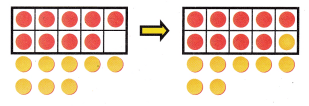(A) 9 + 2 + 8
(B) 9 + 1 + 7
(C) 10 + 1 + 8